## Wednesday, January 27, 2016

### Multi-dimensional Knapsack: Genetic Algorithm vs MIP

In http://pyeasyga.readthedocs.org/en/latest/examples.html a small example of a knapsack problem is shown. Here it has two constraints instead of the usual one (hence multi-dimensional). Note that we are maximizing the objective. The code to solve this with a genetic algorithm is: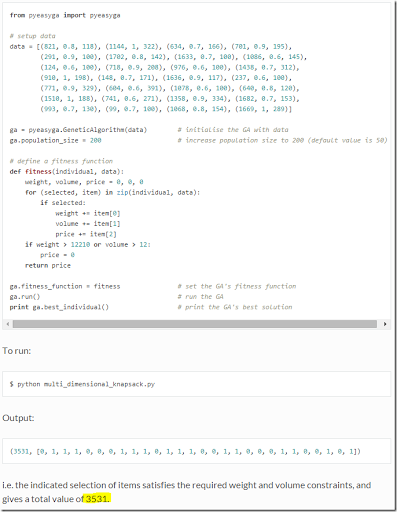When I run it I actually get a somewhat worse objective: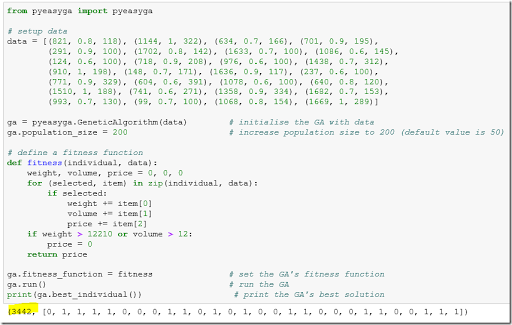When solving the same problem as a MIP: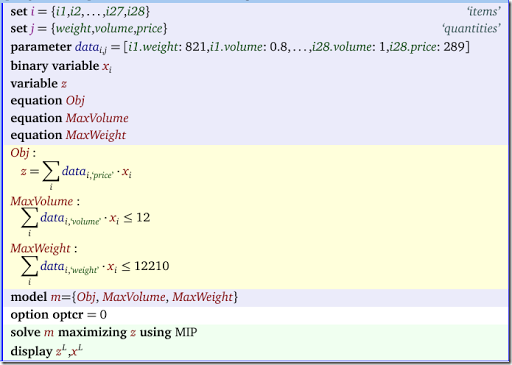we get as solution: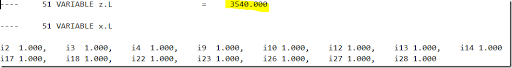Notes:

• Even for some really small examples from the documentation, meta-heuristics find sub-optimal solutions.
• I often see something like: “problem is NP-hard so we must use a meta-heuristic.” I don’t think this is always true.
• MIP solvers have the advantage that they provide optimal solutions for small instances. For large difficult problems they can give a good solution and in addition a measure of how good this solution is (the gap).  Meta-heuristics do not have this property.
• Some commercial MIP solvers actually use heuristics extensively inside their algorithms to help find good solutions quickly.
• I have implemented a number of heuristic algorithms for some nasty problems, but I always try to start with a MIP implementation to get a baseline (both wrt performance and solution qualities).

## Friday, January 22, 2016

### ggplot example

To produce high-quality plots for a document R’s ggplot is always a good choice:

 ```> library(ggplot2) > df<- read.csv("c:\\tmp\\testsolution.csv") > head(df) ID Performance Cost Weight 1 1 0.7051 4365766 49595 2 2 0.7071 4366262 50335 3 3 0.7091 4367526 51091 4 4 0.7110 4368147 50242 5 5 0.7130 4369411 50998 6 6 0.7149 4372412 53125 > ggplot(data=df,aes(x=Cost,y=Performance))+geom_point(aes(color=Weight))+scale_color_gradientn(colors = rainbow(5)) > ggplot(data=df,aes(x=Weight,y=Performance))+geom_point(aes(color=Cost))+scale_color_gradientn(colors = rainbow(5))```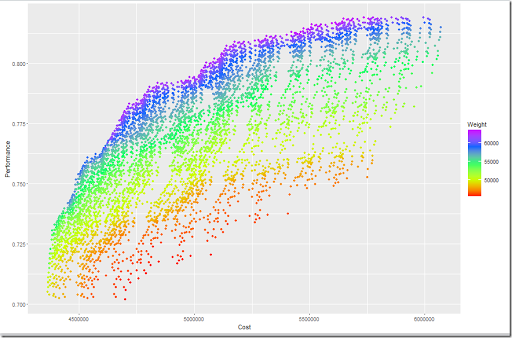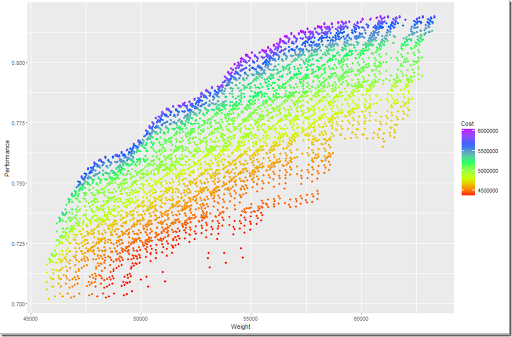##### Update

After some fine tuning:

• Reverse coloring scheme (red=bad, i.e. heavy or expensive)
• Add line from Utopia point to Compromise point (smallest normalized distance)
• Longer legend

 ```> library(ggplot2) > df<- read.csv("c:\\tmp\\testsolution.csv") > # > # Utopia Point > # > U<-data.frame("Cost"=min(df\$Cost), + "Weight"=min(df\$Weight), + "Performance"=max(df\$Performance)) > # > # Ranges > # > R<-data.frame("Cost"=max(df\$Cost)-min(df\$Cost), + "Weight"=max(df\$Weight)-min(df\$Weight), + "Performance"=max(df\$Performance)-min(df\$Performance)) > # > # add column to df with distance from Utopia point > # > df\$Distance = sqrt( + ((df\$Cost-U\$Cost)/R\$Cost)^2+ + ((df\$Weight-U\$Weight)/R\$Weight)^2+ + ((df\$Performance-U\$Performance)/R\$Performance)^2 + ) > # > # Compromise point > # > mindist = min(df\$Distance) > C=df[df\$Distance==mindist,] > > > ggplot(data=df,aes(x=Cost,y=Performance))+ + geom_point(aes(color=Weight))+ + scale_color_gradientn(colors = rev(rainbow(5)))+ + ggtitle("testsolution.csv")+ + theme(legend.key.height=unit(4, "line"))+ + annotate("point",x=U\$Cost,y=U\$Performance,size=3,color='black')+ + annotate("segment",x=C\$Cost,y=C\$Performance,xend=U\$Cost,yend=U\$Performance) > ggplot(data=df,aes(x=Weight,y=Performance))+ + geom_point(aes(color=Cost))+ + scale_color_gradientn(colors = rev(rainbow(5)))+ + ggtitle("testsolution.csv")+ + theme(legend.key.height=unit(4, "line"))+ + annotate("point",x=U\$Weight,y=U\$Performance,size=3,color='black')+ + annotate("segment",x=C\$Weight,y=C\$Performance,xend=U\$Weight,yend=U\$Performance)```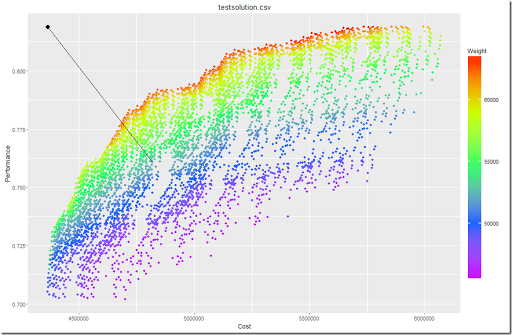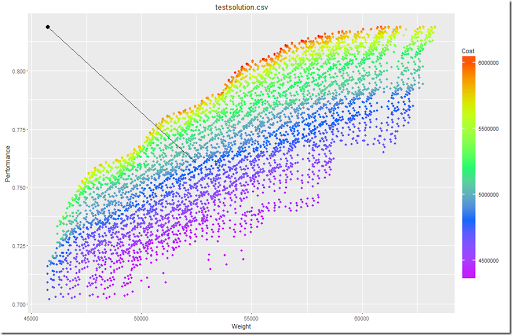Another interesting picture is to color by distance: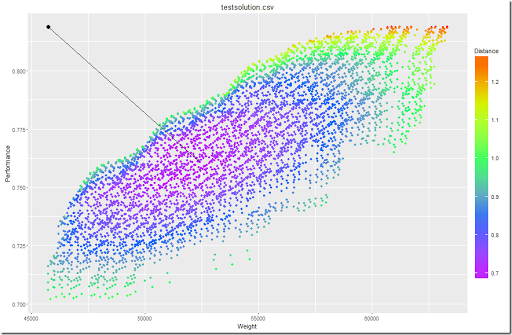## Wednesday, January 20, 2016

### Dataframe Pivoting: some timings

In Pivot a table: GAMS, SQLite, R and Python we described a pivoting operation on a small example. In the comments it was mentioned that for larger tables or dataframes, especially tydir is very fast. Here we try to confirm this using an artificial example: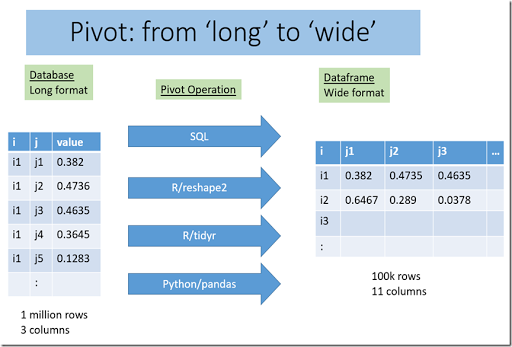GAMS Step Code Time (seconds) GAMS populate a 2D parameter with 1 million entries set i /i1*i100000/    j /j1*j10/;parameter p(i,j);p(i,j) = uniform(0,1); 0.4 Write a GDX file execute_unload 'x.gdx'; 0.3 Store in SQLite database execute 'gdx2sqlite -i x.gdx -o x.db -fast'; 5 R Step Code Time (seconds) Read database table `df1<-dbGetQuery(db,"select * from p")` 2 Pivot using SQL (no indices) ```df2<- dbGetQuery(db,"select tj1.i as i, tj1.value as j1, tj2.value as j2, + tj3.value as j3, tj4.value as j4, tj5.value as j5, + tj6.value as j6, tj7.value as j7, tj8.value as j8, + tj9.value as j9, tj10.value as j10 + from (select i,value from p where j='j1') as tj1, + (select i,value from p where j='j2') as tj2, + (select i,value from p where j='j3') as tj3, + (select i,value from p where j='j4') as tj4, + (select i,value from p where j='j5') as tj5, + (select i,value from p where j='j6') as tj6, + (select i,value from p where j='j7') as tj7, + (select i,value from p where j='j8') as tj8, + (select i,value from p where j='j9') as tj9, + (select i,value from p where j='j10') as tj10 + where tj1.i=tj2.i and tj1.i=tj3.i and tj1.i=tj4.i and tj1.i=tj5.i + and tj1.i=tj6.i and tj1.i=tj7.i and tj1.i=tj8.i + and tj1.i=tj9.i and tj1.i=tj10.i")``` 50 Pivot using SQL after creating indices on columns i and j `id (same query as above)` 15 Pivot using SQL (no indices, alternative query) ```> df1 <- dbGetQuery(db," + select i, + sum(case when j='j1' then value else null end) as j1, + sum(case when j='j2' then value else null end) as j2, + sum(case when j='j3' then value else null end) as j3, + sum(case when j='j4' then value else null end) as j4, + sum(case when j='j5' then value else null end) as j5, + sum(case when j='j6' then value else null end) as j6, + sum(case when j='j7' then value else null end) as j7, + sum(case when j='j8' then value else null end) as j8, + sum(case when j='j9' then value else null end) as j9, + sum(case when j='j10' then value else null end) as j10 + from p + group by i")``` 5 Pivot using reshape2/dcast `df2<-dcast(df1,i~j,value.var="value")` 2 Pivot using tidyr/spread `df2<-spread(df1,j,value)` 2 Python Step Code Time (seconds) pandas/pivot df2=df1.pivot(index='i',columns='j',values='value') 2
##### Conclusion

The methods that work on in-memory datastructures are faster than SQL but depending on the SQL formulation we can come close.  The big advantage of the pivot methods in R and Python is that they require much less typing (and less thought).

## Tuesday, January 19, 2016

### SciPy infeasibility error

This model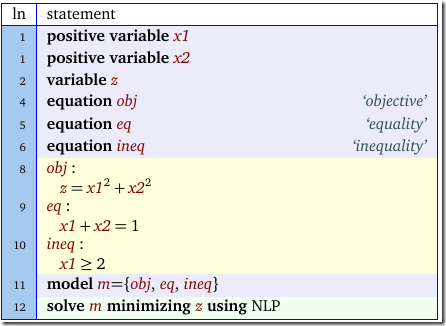is obviously infeasible. However SciPy’s SLSQP algorithm returns as if everythin is hunky-dory:(I hope I did not make a mistake here: things are not as readible as I would like). R also has an interface to SLSQP. Here we see some flags being raised:

```> slsqp(c(1,2),
+       function(x) {x^2+x^2},
+       heq=function(x){x+x-1},
+       hin=function(x){x-2},
+       lower=c(0,0))
\$par
 1.666667e+00 4.773719e-11

\$value
 2.777778

\$iter
 105

\$convergence
 -4

\$message
 "NLOPT_ROUNDOFF_LIMITED: Roundoff errors led to a breakdown of the optimization algorithm. In this case, the returned minimum may still be useful. (e.g. this error occurs in NEWUOA if one tries to achieve a tolerance too close to machine precision.)"```

## Sunday, January 17, 2016

### Cutting Wood using Optimization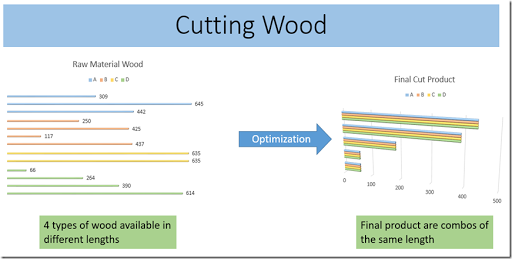In this post an interesting problem was described:

• Raw material is a number of lengths of wood types A,B,C and D.
• We want to cut it so we can create final product. This final product consists of combos of wood types A,B,C and D of the same length.
• The final product can not be shorter than 30.
• In the post it was requested to produce a cutting scheme such that left over raw material of length less than 30 (waste) is minimized.

If we would just use this objective (minimize waste) the optimal strategy would be “don’t cut anything”. Therefore our first approach is to use a slightly different objective: create as much final product as we can. Later on we will discuss some refinements.

As usual there is more than one way to skin a cat, here is just one way of doing that. First introduce our basic “assignment” variables and the index sets they operate upon: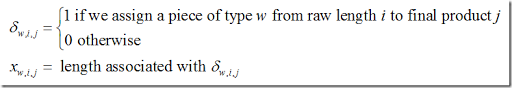The assignment structure follows from these fundamentals.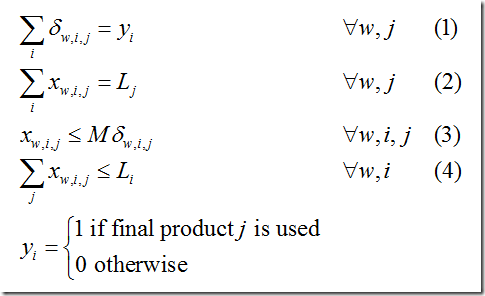1. The first equation is used to make sure that exactly one raw piece is used for each wood type in a final product. We introduce a binary variable yj to keep track which j’s are actually created (typically we have more j’s in the model than needed: we don’t know this number in advance).
2. Equation (2) deals with the length of the final product combo. Note that Lj does not depend on the wood type w. So all pieces in the combo have the same length. Note that this final product combo length is actually a decision variable.
3. Inequality (3) simply makes sure δw,i,j=0 ⇒ xw,i,j=0.
4. Finally inequality (4) deals with raw material lengths. This Li is a constant.

The data set we will use is: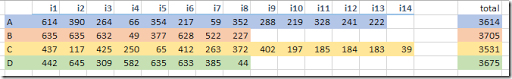This means in the model we do not want to use all combinations (w,i) but rather a subset wi(w,i). A complete model setup can look like: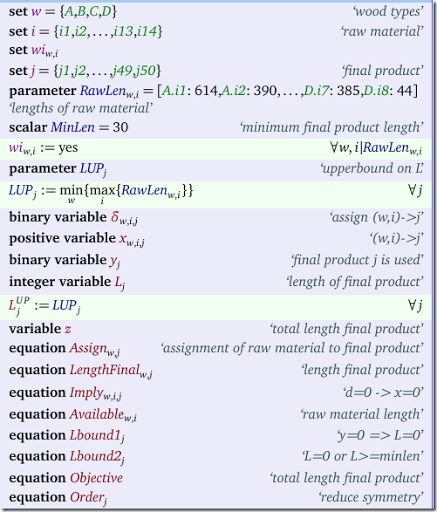The model equations are: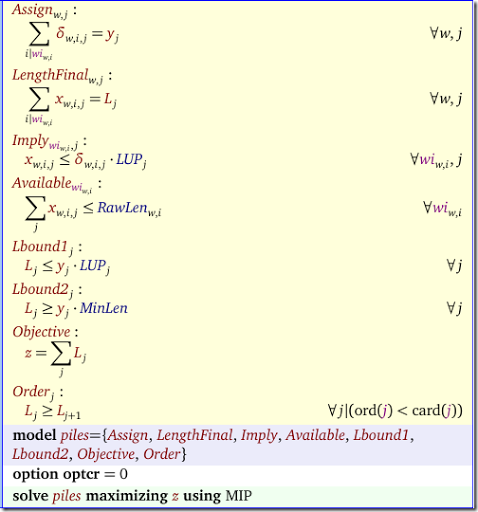Notes:

• The variables Lj are declared integer, just to get nicer solutions.
• The order equation helps producing nicer reports but also helps in speeding up things.
• We solved with Cplex with some extra options:
• Threads to allow parallel B&B
• Mipemphasis to indicate we want to go to optimality
• Priorities on the discrete variables to help Cplex a bit
• The solution time was < 2 minutes (to proven optimality).

The final results look like: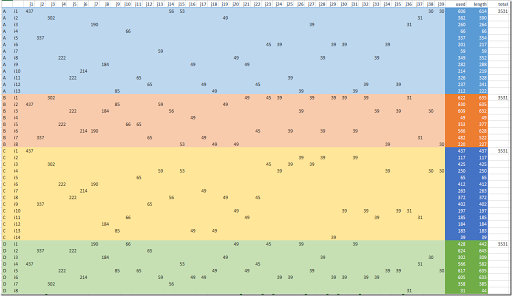The rows represent the raw material and the columns are the final product combos. Each column should have exactly four entries (one from each color or wood type). The columns are ordered by length and the smallest length is 30. On the right we verify that we have never exceeded the raw material length. In this solution we have used up all the yellow (wood type C) raw material.

##### Objective

The proposed objective in the original post is:

(1) Minimize Waste

where waste is left over cuts with a length < 30. The problem with this objective is that “doing nothing” would be an optimal solution.

In our model we used the objective:

(2) Maximize total length of final product

Actually this is not such a bad choice. We can see we use up the yellow wood type C (this has a smallest amount of raw material) without any waste.

If we know the demand for the final product we can use the following model:

(3) Minimize Waste
Subject to meeting demand

If we don’t know demand in advance and the final product is made for inventory, we can use a different approach. We assign dollar values to both raw material lengths and final product lengths. Of course a raw material length length of < 30 gets a zero value (or may be a small scrap value). Now we can do:

(4) Maximize Total Value

We can refine this further by assigning different values to different lengths, so we can favor longer lengths.

## Friday, January 15, 2016

### Pivoting a table: GAMS, SQLite, R and Python

In an earlier post I read a GAMS variable into Python program. Here I add some more notes on the tools and procedures we can use for this.

In this example we want to convert a long table coming from a GAMS model, into a wide dataframe for further processing in R or Python.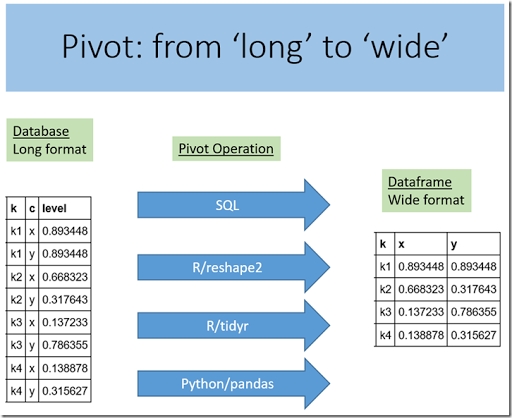This operation is not very unusual. With the right tools, it is not so difficult to perform this task.

##### 1. GAMS: Export to GDX and dump into SQLite

We are interested in variable loc which is declared as:

positive variable loc(k,c) 'location of sensor';

After the solve we do a display of loc.L (i.e. the levels) and we see:

 ----     36 VARIABLE loc.L  location of sensor              x           y k1       0.893       0.893k2       0.668       0.318k3       0.137       0.786k4       0.139       0.316

The data is displayed here as a matrix. We can also display it in a list format, which resembles more how the data will be stored in a GDX file or a typical “long” database table:

 ----     39 VARIABLE loc.L  location of sensor k1.x 0.893k1.y 0.893k2.x 0.668k2.y 0.318k3.x 0.137k3.y 0.786k4.x 0.139k4.y 0.316

Here we just use two different display styles to show the same data. Later on we will see that data can also be actually stored in these two formats. This applies to database tables and R or Python dataframes.

To store the loc variable in an SQLite database we can use the following in the GAMS model:

execute 'gdx2sqlite -i results.gdx -o results.db -fast'
;

As we will see below, the variable does not only store the level but also the lower and upperbound and the marginal.
##### 2. SQLite in R: RSQLite

In R we can use the RSQLite package to read data from SQLite databases:

`> library(RSQLite) `
`Loading required package: DBI`

> sqlite<-dbDriver("SQLite")
>
db <- dbConnect(sqlite,"c:\\projects\\tmp\\code\\results.db")
> dbListTables(db)

 "c"               "cov"             "covk"

 "i"               "iscovered"       "iscoveredk"

 "j"               "k"               "loc"

 "scalars"         "scalarvariables"

> dbGetQuery(db,"select * from loc")

k c     level lo up      marginal

1 k1 x 0.8934478  0  1  1.942971e-12

2 k1 y 0.8934479  0  1  1.942972e-12

3 k2 x 0.6683234  0  1 -3.541285e-13

4 k2 y 0.3176426  0  1 -7.418214e-13

5 k3 x 0.1372328  0  1 -1.447327e-12

6 k3 y 0.7863548  0  1 -3.002015e-13

7 k4 x 0.1388778  0  1 -1.699826e-12

8 k4 y 0.3156270  0  1 -7.479254e-13

Note that the table and resulting dataframe are in “long” format. To select the x and y coordinates we need to do something like:

`> x <- dbGetQuery(db,"select k,level as x `
`+                     from loc `
`+                     where c='x'")`
`> y <- dbGetQuery(db,"select k,level as y `
`+                     from loc `
`+                     where c='y'")`
`> x`
`   k         x`
`1 k1 0.8934478`
`2 k2 0.6683234`
`3 k3 0.1372328`
`4 k4 0.1388778`
`> y`
`   k         y`
`1 k1 0.8934479`
`2 k2 0.3176426`
`3 k3 0.7863548`
`4 k4 0.3156270`
In the following sections we will try to get x and y as columns in one single dataframe.
##### 3. Pivot in SQL

A big advantage of SQL is that we can do some data manipulation steps before creating and populating the dataframe. In this case we want to do a pivot operation where the level column becomes two columns x and y. Of course we can do that in SQL. There are many ways to implement this, and here is an example:

`> dbGetQuery(db,"select tx.k as k, tx.level as x, ty.level as y `
`+                from (select k,level from loc where c='x') as tx, `
`+                     (select k,level from loc where c='y') as ty `
`+                where tx.k=ty.k")`
`   k         x         y`
`1 k1 0.8934478 0.8934479`
`2 k2 0.6683234 0.3176426`
`3 k3 0.1372328 0.7863548`
`4 k4 0.1388778 0.3156270`

A simpler and faster SQL query is:

```> dbGetQuery(db,"
+   select k,
+          sum(case when c='x' then level else null end) as x,
+          sum(case when c='y' then level else null end) as y
+   from loc
+   group by k")
k         x         y
1 k1 0.8934478 0.8934479
2 k2 0.6683234 0.3176426
3 k3 0.1372328 0.7863548
4 k4 0.1388778 0.3156270```

Note: There is an SQL PIVOT construct. As of now it seems supported only by SQL Server and Oracle.

##### 4. Pivot in R: reshape2

The pivot operation can also be performed in R. Here we use the dcast function of the reshape2 package:

`> library(reshape2)`
`> df1<-dbGetQuery(db,"select k,c,level from loc")`
`> df1`
`   k c     level`
`1 k1 x 0.8934478`
`2 k1 y 0.8934479`
`3 k2 x 0.6683234`
`4 k2 y 0.3176426`
`5 k3 x 0.1372328`
`6 k3 y 0.7863548`
`7 k4 x 0.1388778`
`8 k4 y 0.3156270`
`> df2<-dcast(df1,k~c,value.var="level")`
`> df2`
`   k         x         y`
`1 k1 0.8934478 0.8934479`
`2 k2 0.6683234 0.3176426`
`3 k3 0.1372328 0.7863548`
`4 k4 0.1388778 0.3156270`
##### 5. Pivot in R: tidyr

The tidyr package is a successor to reshape2. The function we use to pivot our data is called spread:

`> library(tidyr)`
`> df1<-dbGetQuery(db,"select k,c,level from loc")`
`> df1`
`   k c     level`
`1 k1 x 0.8934478`
`2 k1 y 0.8934479`
`3 k2 x 0.6683234`
`4 k2 y 0.3176426`
`5 k3 x 0.1372328`
`6 k3 y 0.7863548`
`7 k4 x 0.1388778`
`8 k4 y 0.3156270`
`> spread(df1,c,level)`
`   k         x         y`
`1 k1 0.8934478 0.8934479`
`2 k2 0.6683234 0.3176426`
`3 k3 0.1372328 0.7863548`
`4 k4 0.1388778 0.3156270`

##### 6. SQLite in Python

In Python we use packages SQLite3 and of course pandas.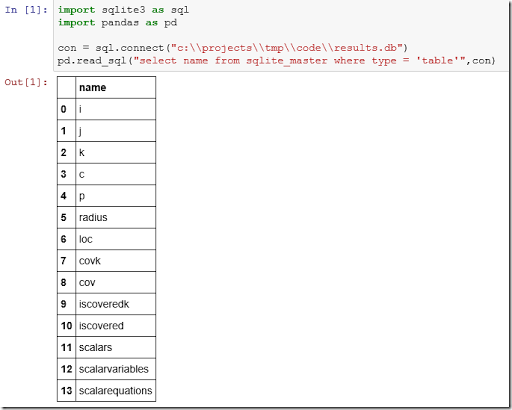There is no built-in “list tables” function so we use the master table of the SQLite database. The table loc looks like: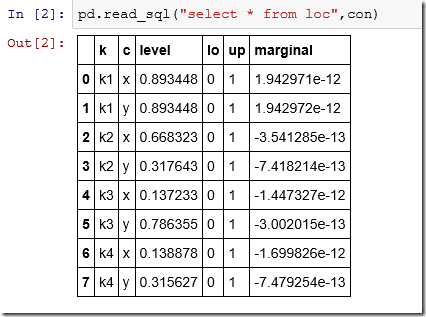The SQL “pivot” query is long so we can use a multi-line string:

##### 7. Pivot in Python: pandas.pivot

Pandas has a sophisticated pivot method: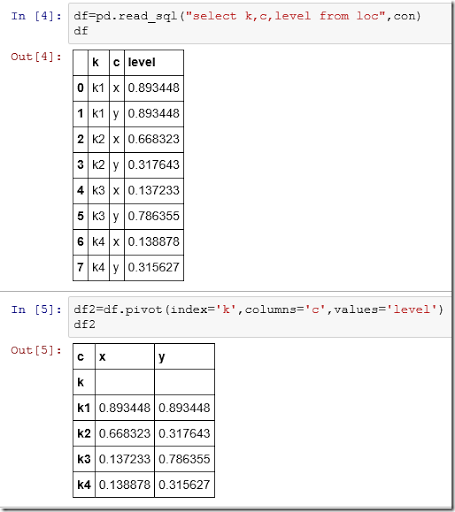The output is not exactly the same as with the SQL approach, but it is close. We just need to do one more step to make the index labels k a normal data column: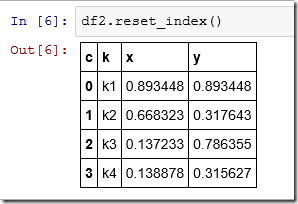##### Conclusion

When we move data from GAMS –> SQLite –> dataframe, we often need to make some data manipulation steps, some of which may not be totally trivial. But we have powerful and flexible tools at our disposal: SQL and R/Python. In this case we showed that a pivot operation to transform a “long” table into a “wide” one can be done either at the SQL level when importing the data into R/Python or directly in R/Python.

##### Update

Some timings on a larger data set are here.